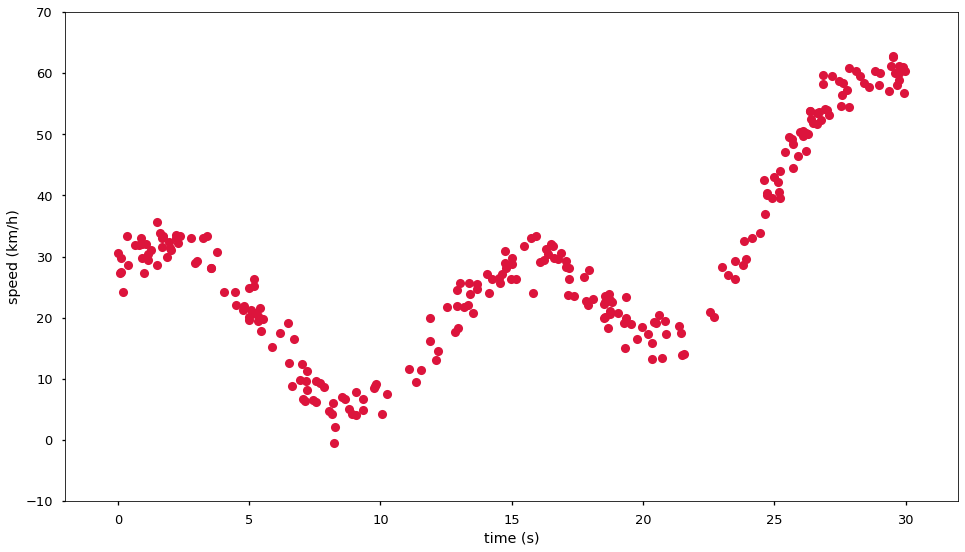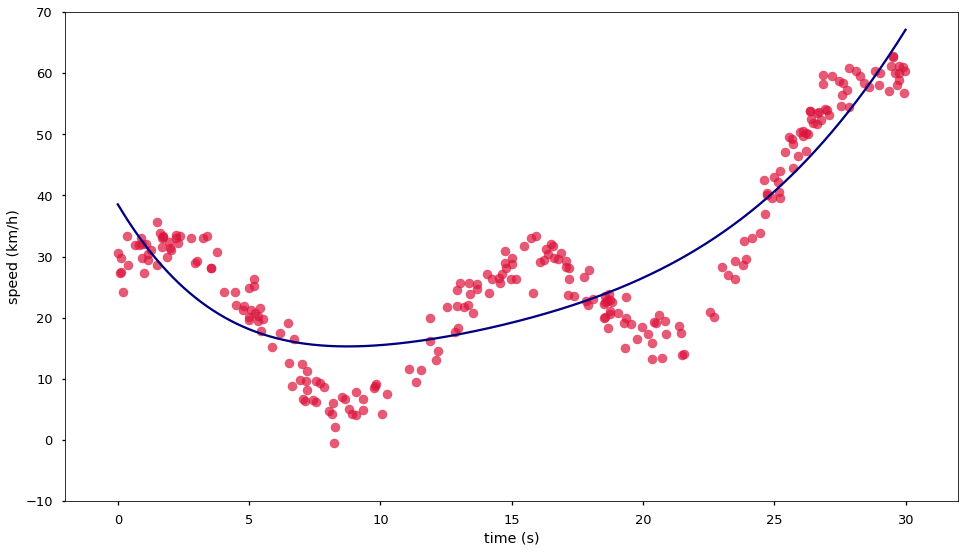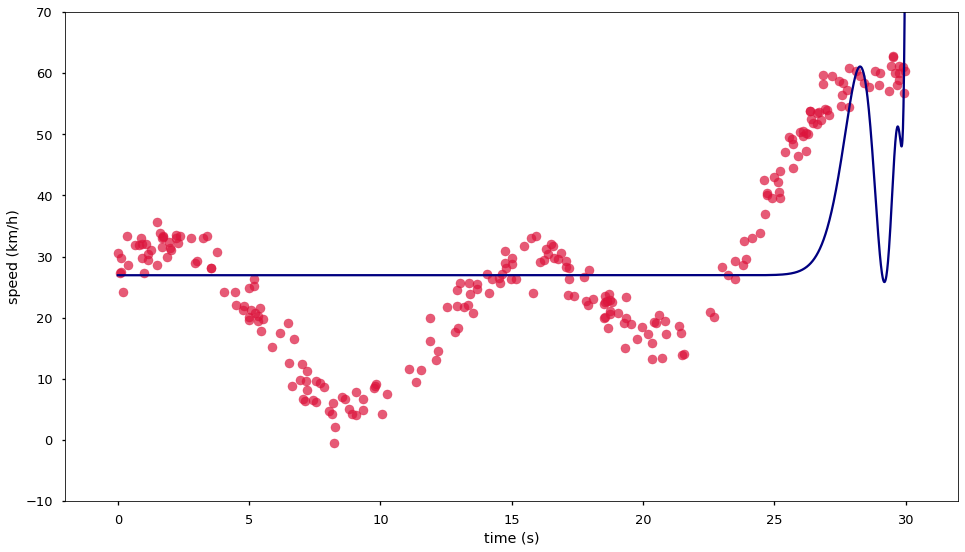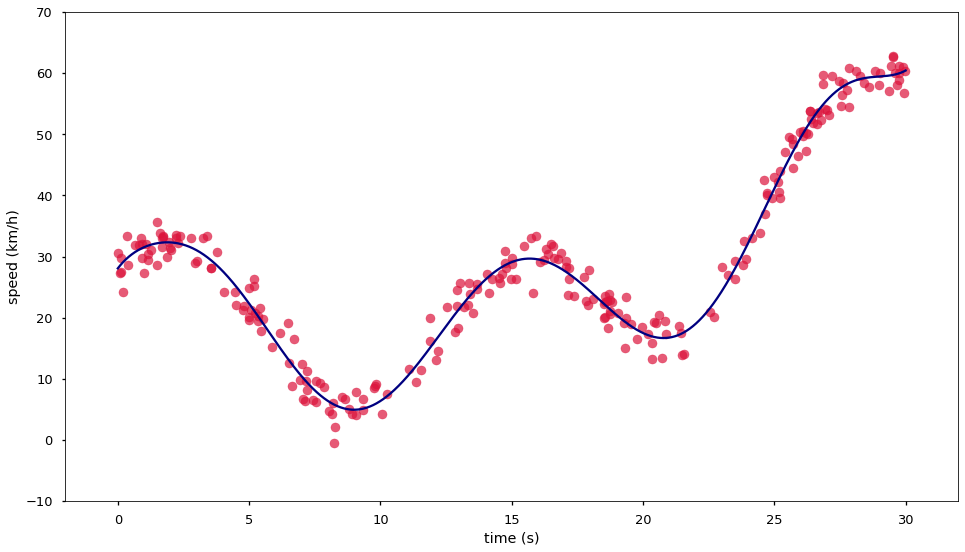Let's move forward: can we estimate the speed at each point on a longer strip of a road? For example, some 30km of a road with turns and inclines.fe-formula-one.svg

We import the common suspects and make a short simulation of a road trip.

In :
import numpy as np
import matplotlib.pyplot as plt
%matplotlib inline
plt.style.use('seaborn-talk')
from sklearn.linear_model import LinearRegression
from sklearn.preprocessing import PolynomialFeatures
from sklearn.pipeline import make_pipeline


For our simulation it is interesting to note that most problems in the real world are not linear, they are either exponential or periodic. Why? Something, something, complexity theory. Anyway, speed on a road is a periodic problem, one speeds up and down in response to the shape of the road he drives on.

In :
t = 30*np.random.rand(256)
spd = 13*np.sin(t/2) + 3.7*np.cos(t/2+7) + 3*t + 0.1*(t-10)**2 - 3*(t-3) + 7 + 2.3*np.random.randn(*t.shape)
fig, ax = plt.subplots(figsize=(16, 9))
ax.plot(t, spd, 'o', color='crimson')
ax.set(xlabel='time (s)', ylabel='speed (km/h)', xlim=(-2, 32), ylim=(-10, 70));It is pretty difficult to figure out what polynomial degree we need for this fit. But let's try a guess, degree 5.

For the time being we will not worry about a test set or cross validation, we will just explore the data. Once we know something about the problem we will come back and perform proper model evaluation.

In :
model = make_pipeline(PolynomialFeatures(degree=5), LinearRegression())
model.fit(t[:, np.newaxis], spd[:, np.newaxis])
xfit = np.linspace(0, 30, 3000)
yfit = model.predict(xfit[:, np.newaxis])
fig, ax = plt.subplots(figsize=(16, 9))
ax.scatter(t, spd, color='crimson', alpha=0.7)
ax.plot(xfit, yfit, color='navy')
ax.set(xlabel='time (s)', ylabel='speed (km/h)', xlim=(-2, 32), ylim=(-10, 70))
model

Out:
Pipeline(memory=None,
steps=[('polynomialfeatures',
PolynomialFeatures(degree=5, include_bias=True,
interaction_only=False, order='C')),
('linearregression',
LinearRegression(copy_X=True, fit_intercept=True, n_jobs=None,
normalize=False))],
verbose=False)Ouch, that went pretty badly. This is a case where out model underfits the data, i.e. our model has not enough complexity to model the complexity we see. We can also say that our model has too much bias about how the data looks.

Let us try with a big degree, e.g. 100.

In :
model = make_pipeline(PolynomialFeatures(degree=100), LinearRegression())
model.fit(t[:, np.newaxis], spd[:, np.newaxis])
xfit = np.linspace(0, 30, 3000)
yfit = model.predict(xfit[:, np.newaxis])
fig, ax = plt.subplots(figsize=(16, 9))
ax.scatter(t, spd, color='crimson', alpha=0.7)
ax.plot(xfit, yfit, color='navy')
ax.set(xlabel='time (s)', ylabel='speed (km/h)', xlim=(-2, 32), ylim=(-10, 70))
model

Out:
Pipeline(memory=None,
steps=[('polynomialfeatures',
PolynomialFeatures(degree=100, include_bias=True,
interaction_only=False, order='C')),
('linearregression',
LinearRegression(copy_X=True, fit_intercept=True, n_jobs=None,
normalize=False))],
verbose=False)That ain't good either. On the left hand side we passed the point where we can bend the polynomial and our parameters mess with each other. On the right hand side the polynomial overfits the data; or we say that the model has too much variance. There are two different examples of overfitting on the graph.

On the right we see a classical case where the function has too much variance. In such a case the parameters will attempt to move the function directly through every single point, and fail to generate a reasonable function across the points in pretty much all cases.

On the left the linear regression did attempt to find good parameters for several degrees but every time it increased a parameter to, say, $t^{36}$ in order to make the function go up in a specific place it did affect the other places around the function. In turn the function attempted to change the parameter for, say, $t^{47}$ in order to counter the problems generated by the previous parameter increase but this again backfired by changing the function value in other places. The issue happens because linear regression assumes that the is no relationship between the different variables to which the parameters are multiplied. But here $t^{36}$ is very related to $t^{47}$, and also to $t^{63}$, and to pretty much every other value from polynomial features.

There is more than one way to solve this problem. Let's see one way: model selection.

## Bias versus Variance - Model Selection¶

To solve the right hand side problem we need to tune of hyperparameter, the degree of our polynomial. And then we hope that the left hand side get solved due to a smaller number of parameters. In the case where the left hand side does not get solved then we need to look into regularization - which we will see next.

Until now we have been guessing and doing this by hand but trying all values between $5$ and $100$ by hand does not seem like a good way of spending an afternoon. Instead sklearn can automate this for us.

We will train a model for every degree between $5$ and $100$ and evaluate, by cross-validation, which model performs better. sklearn provides us with a grid search algorithm, which will perform the training and cross-validating of our model for all hyperparameter values given to it. The GridSearchCV is another sklearn object that takes sklearn objects and gives out a similar interface. When one performs fit on a grid search object, it will train models with all possible combinations given in the grid of hyperparameters. It then performs cross validation on every model and chooses the model which has the best mean cross validation score. The cross validation argument we use cv=5 is the sklearn's default. It performs $5$ fold splits without shuffling the data, in order to perform a shuffle one can use a KFold in the same fashion as with cross_val_score.

Here we define the grid search to train $95$ linear regression models, each with a different value for the degree of the polynomial features given to the regression itself. We give into the grid search a model that itself is a pipeline of models. That is no issue for the grid search, note how can we specify the element of the pipeline to which the hyperparameter range is to be applied by using a double underscore. We use polynomialfeatures__degree to refer to the degree= hyperparameter of the PolynomialFeatures object, inside the pipeline. And the pipeline itself allows for more shortcuts to the contained objects with the named_steps attribute.

We are starting to automate hyperparameter selection. Once a best set of hyperparameters is identified by the grid search, it trains a model with these hyperparameter values and all the data. This trained model is then available under best_estimator_, and the cross validation mean score that allowed the selection of this model as best under best_score_

In :
from sklearn.model_selection import GridSearchCV

model = make_pipeline(PolynomialFeatures(degree=5), LinearRegression())
grid = GridSearchCV(model,
{'polynomialfeatures__degree': list(range(5, 101))},
cv=5)
grid.fit(t[:, np.newaxis], spd[:, np.newaxis])
best = grid.best_estimator_

xfit = np.linspace(0, 30, 3000)
yfit = best.predict(xfit[:, np.newaxis])
fig, ax = plt.subplots(figsize=(16, 9))
ax.scatter(t, spd, color='crimson', alpha=0.7)
ax.plot(xfit, yfit, color='navy')
ax.set(xlabel='time (s)', ylabel='speed (km/h)', xlim=(-2, 32), ylim=(-10, 70))
best, best.named_steps.linearregression.intercept_, best.named_steps.linearregression.coef_

Out:
(Pipeline(memory=None,
steps=[('polynomialfeatures',
PolynomialFeatures(degree=10, include_bias=True,
interaction_only=False, order='C')),
('linearregression',
LinearRegression(copy_X=True, fit_intercept=True, n_jobs=None,
normalize=False))],
verbose=False),
array([28.07079204]),
array([[ 0.00000000e+00,  5.63557642e+00, -2.98208857e+00,
1.08366384e+00, -3.23484837e-01,  5.24801162e-02,
-4.64249930e-03,  2.34704276e-04, -6.79413019e-06,
1.04914400e-07, -6.70557831e-10]]))And we should have a look at the R2 of the best estimator we have built.

In :
grid.best_score_

Out:
0.9766121925237712

Often simple hyperparameter tuning is enough to solve even complex problems. Yet, the problem we saw on the left hand side was a problem of dependence between dimensions, and sometimes it cannot be easily solved. We shall look at more techniques.